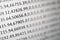## Applications of the bootstrap techniques in RPhoto by Mika Baumeister on Unsplash

Every measure must be followed by an error estimate. There’s no chance to avoid this. If I tell you “I’m 1,93 metres tall”, I’m not giving you any information about the precision of this measure. You could think that my precision is on the second decimal digit, but you can’t be sure.

So, what we really need is some way to assess the precision of our measure starting from the data sample we have.

If our observable is the mean value calculated over a sample, a simple precision estimate is given by the standard error. But what can we do if we are measuring something that is not the mean value? That’s the point at which bootstrap comes in help.

# Bootstrap in a nutshell

Bootstrap is a technique made in order to measure confidence intervals and/or standard error of an observable that can be calculated on a sample.

It relies on the concept of resampling, which is a procedure that, starting from a data sample, simulates a new sample of the same size, considering every original value with replacement. Each value is taken at the same probability of the others (which is 1/N).

So, for example, if our sample is made by the four values {1,2,3,4}, some possible resampling can be {1,4,1,3}, {1,2,4,4}, {1,4,3,2} and so on. As you can see, the values are the same, they can be repeated and the samples have the same size as the original one.

Now, let’s say we have a sample of N values and want to calculate some observable O, that is a function that takes in input all the N values and gives in output one real number. An example can be the average value, the standard deviation or even more complex functions as the quantiles, the Sharpe ratio and so on.

Our goal is to calculate the expected value of this observable and, for example, its standard error.

To accomplish this goal, we can run the resampling procedure M times and, for each sample, we can calculate our observable. At the end of the process, we have M different values of O, that can be used to calculate the expected value, the standard error, the confidence intervals and so on.

This simple procedure is the bootstrap process.

# An example in R

R has a wonderful library called bootstrap that performs all the calculations for us.

Let’s say we want to measure the skewness of the sepal length in the famous iris dataset and let’s say we want to know its expected value, its standard error and the 5th and 9th percentile.

Here follows the R code that makes this possible:

The mean value is 0.29, the standard deviation is 0.13, the 5th percentile is 0.10 and the 95th percentile is 0.49.

These numbers allow us to say that the real skewness value of the dataset is 0.29 +/- 0.13 and it is between 0.10 and 0.49 with a 90% confidence.

Surprisingly, isn’t it?

This simple procedure is universal and can be used with any kind of observable.

# The larger the dataset, the higher the precision

Somebody could think that the larger our dataset, the smaller the standard error we take due to the law of large number.

It’s definitely true and we can simulate this case.

Let’s simulate N random uniformly distributed numbers and let’s calculate the skewness over them. What we expect is that the standard deviation of the bootstrapped skewness decreases as long as N increases.

The following R code performs this calculation and, (not so) surprisingly, the bootstrap standard deviation decreases as a power law with the exponent equal to -1/2.

The slope of the double-log linear regression is the exponent of the power law and it’s equal to -0.5.

# Conclusions

Bootstrap is a method to extract as much information as possible from a finite size dataset and it makes us calculate the expected value of any observable and its precision (i.e. its standard deviation or confidence intervals).

It’s really useful when we need to calculate an error estimate for some scientific measure and it can easily generalized for multivariate observables.

Any data scientist should not forget to use this powerful tool, which has shown several useful application even in machine learning (e.g in the Random Forest classification/regression models).

Written by

## Data Science Journal

#### Articles about data science, mathine learning, artificial intelligence and their impact in our life.

Welcome to a place where words matter. On Medium, smart voices and original ideas take center stage - with no ads in sight. Watch
Follow all the topics you care about, and we’ll deliver the best stories for you to your homepage and inbox. Explore
Get unlimited access to the best stories on Medium — and support writers while you’re at it. Just \$5/month. Upgrade